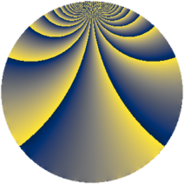# Properties

 Label 572.2.sLevel $572$ Weight $2$ Character orbit 572.s Rep. character $\chi_{572}(43,\cdot)$ Character field $\Q(\zeta_{6})$ Dimension $160$ Newform subspaces $1$ Sturm bound $168$ Trace bound $0$

# Related objects

## Defining parameters

 Level: $$N$$ $$=$$ $$572 = 2^{2} \cdot 11 \cdot 13$$ Weight: $$k$$ $$=$$ $$2$$ Character orbit: $$[\chi]$$ $$=$$ 572.s (of order $$6$$ and degree $$2$$) Character conductor: $$\operatorname{cond}(\chi)$$ $$=$$ $$572$$ Character field: $$\Q(\zeta_{6})$$ Newform subspaces: $$1$$ Sturm bound: $$168$$ Trace bound: $$0$$

## Dimensions

The following table gives the dimensions of various subspaces of $$M_{2}(572, [\chi])$$.

Total New Old
Modular forms 176 176 0
Cusp forms 160 160 0
Eisenstein series 16 16 0

## Trace form

 $$160q - 2q^{4} + 68q^{9} + O(q^{10})$$ $$160q - 2q^{4} + 68q^{9} - 36q^{12} - 8q^{14} - 6q^{16} + 30q^{20} - 20q^{22} - 144q^{25} - 40q^{26} - 6q^{33} + 46q^{36} - 12q^{37} - 56q^{38} - 2q^{42} + 48q^{45} - 40q^{48} + 44q^{49} - 32q^{53} + 30q^{56} + 78q^{58} + 64q^{64} - 4q^{66} - 44q^{69} - 44q^{77} + 16q^{78} + 24q^{80} - 40q^{81} - 6q^{82} - 10q^{88} + 24q^{89} - 24q^{92} - 96q^{93} + O(q^{100})$$

## Decomposition of $$S_{2}^{\mathrm{new}}(572, [\chi])$$ into newform subspaces

Label Dim. $$A$$ Field CM Traces $q$-expansion
$$a_2$$ $$a_3$$ $$a_5$$ $$a_7$$
572.2.s.a $$160$$ $$4.567$$ None $$0$$ $$0$$ $$0$$ $$0$$